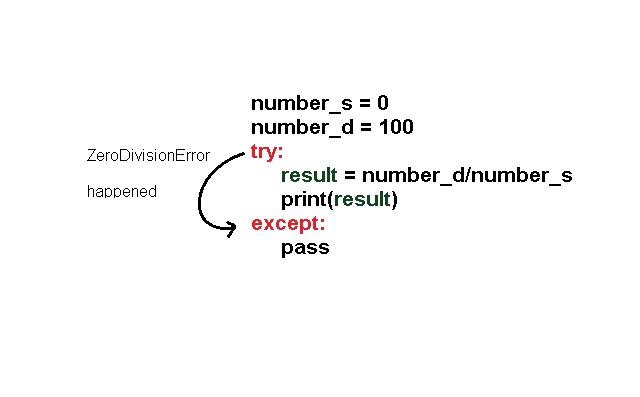# Python Try Block

The python try block is part of python exception handling mechanism. The try block is similar to an if-then-else block. If an error occurs the try block stops executing. These errors are known errors for which we have a try block. They are called exceptions.

The try block is to notice the error or exception that happened and stop executing.

After that you can have a catch block in python that will handle the error in most appropriate way. Usually the error handling routine is written by the programmers.

### Syntax Try Block

There may be code outside the try block and any error in those parts will not be catched. The syntax for try block is given below.

``````# The try block
number_d = 10
number_s = int(input("Enter a number :"))
try:
result = number_d/number_s
print(result)
except:
pass``````

In the example above, the variables – `number_d `and `number_s `are declared outside of the `try block`, therefore, no error will be caught.

If the value of `number_s `is 0, there will be a `ZeroDivisionError` and the program will exit without printing anything because the `except `keyword does not do anything and let the block `pass`.

Therefore, output of the above program will be empty. See the figure below.try-except block

Instead of `pass`, you can print a customized error to indicate what error was caught. Note that we already know that a `ZeroDivisionError` would happen.

Consider the modified program from above.

``````# The try block
number_d = 10
number_s = int(input("Enter a number :"))
try:
result = number_d/number_s
print(result)
except:
print("Cannot divide by Zero. Try different integer")
``````

The output of the above program is following when` number_s` is 0.

``````Enter a number :0
Cannot divide by Zero. Try different integer
>>> ``````

In the next article, we will understand more about exception handling, especially, the `catch block(except).`